Math & Beyond

# What is Dot Situation of Images?

0

 1 Introduction 2 Solved Examples 3 Practice Questions 4 Learning Outcome 5 Frequently Asked Questions (FAQs)

08 January 2021

## Introduction

What is dot situation?

Dot Situation is a very important and scoring topic in nonverbal reasoning aptitude. This section is the assessment and testing of a student's perceptive observation power.

The problems in the dot situation include a group of three or more geometrical figures – normally triangle, square, rectangle, or/and circle having one or more dots put any time inside the bunch.

This bunch is trailed by an arrangement of four option figures each made out of a group of some sort in figures.

Presently, for every dot, we need to watch the region in which it is encased, i.e. to which of the geometrical figures this region is regular. At that point, we search for such a region in the four choices.

When we have discovered it, we repeat the method for different dots, assuming any. The option figure which contains every single such region is the answer.

In this section, we are covering some problems considering different dot situations and will discuss their solution also.

## What is Dot Situation of Images?

The problems on dot situation include a group of three or more geometrical figures – normally triangle, square, rectangle or/and circle having one or more dots put any time inside the bunch. This bunch is trailed by an arrangement of four option figures each made out of a group of some sort in figures.

What is Dot Situation of Images? is mentioned below in the Downloadable PDF.

## Solved Examples on Dot Situation

Example 1: Which of the following figures satisfies the same conditions of placement of dots as in Figure?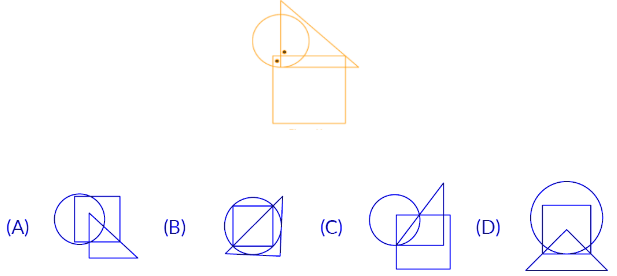Solution:  In the question figure, two dots are given, one dot is common to the circle and triangle region and the second dot is common to the square and circle region, here we have to find out which figure satisfies the same dot situation. Such a dot situation is possible in answer figure (B).

 (B)

Example 2: Select a figure from the options which does not satisfy the same condition of placement of the dots as in Figure.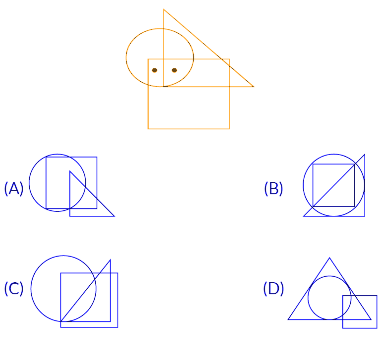Solution:  In the question figure, two dots are given, one dot is common to circle, triangle, and square region. The second dot is common to the square and circle region. Here we have to find out which figure does not satisfy the same dots situation. Such a type of dot situation is not possible in answer figure (D).

 (D)

Example 3: Select the figure which satisfies the same conditions of placement of the dot as in the given figure.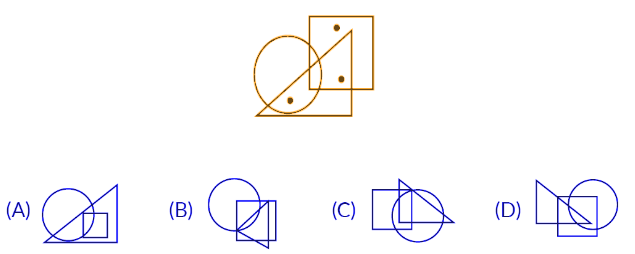Solution: In the question figure, three dots are given, one dot is common to the circle and triangle region, the second dot is common to the square and triangle region and the third dot is present only in the square region. So here we have to find out which answer figure satisfies the same dot situation. Such a dot situation is possible in the answer figure (C).

 (C)

Example 4: Select the figure which satisfies the same conditions of placement of the dot as in the following figure.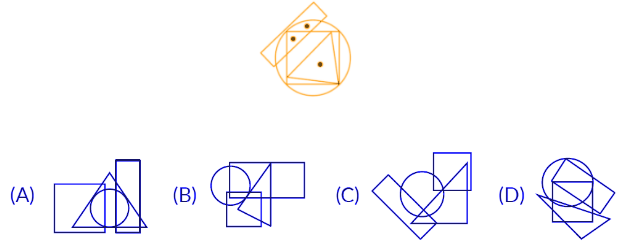Solution: In the question figure, three dots are given, one dot is common to the circle and rectangle region, the second dot is common to the square, triangle and circle region and the third dot is common to triangle, square, and circle. Here we have to find out which answer figure satisfies the same dot situation. Such a dot situation is possible in the answer figure (D).

 (D)

Example 5: Which of the following options satisfies the same condition of placement of dots as in figure?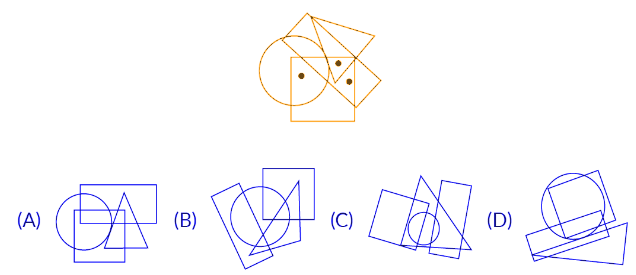Solution: In the question figure, three dots are given, the first dot is common to the circle and square region, the second dot is common to the triangle, square, and rectangle region and the third dot is common to the square and rectangle region only. Here we have to find out which answer figure satisfies the same dot situation. Such a dot situation is possible in the answer figure (A).

 (A)

## Practice Questions

Example 1: Select the correct option in which you will be able to place the dot(s) as per the question figure.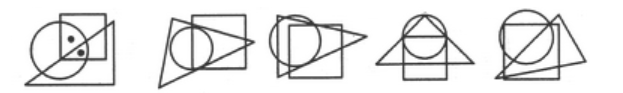Figure (X)              (1)             (2)               (3)                (4)

Options: A. (1)      B. (2)      C. (3)      D. (4)

Example 2 : Select the correct option in which you will be able to place the dot(s) as per the question figure.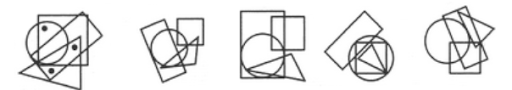Figure (X)              (1)             (2)               (3)                (4)

Options: A. (1)      B. (2)      C. (3)      D. (4)

Example 3 : Select the correct option in which you will be able to place the dot(s) as per the question figure.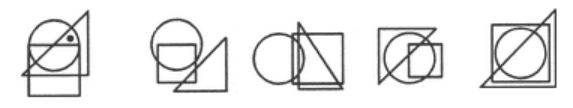Figure (X)              (1)                   (2)                 (3)               (4)

Options: A. (1)      B. (2)      C. (3)      D. (4)

Example 4 : Select the correct option in which you will be able to place the dot(s) as per the question figure.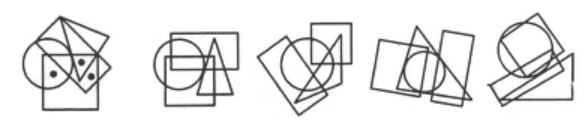Figure (X)              (1)             (2)               (3)                (4)

Options: A. (1)      B. (2)      C. (3)      D. (4)

 1. (D)      2. (D)      3. (C)      4.(A)

## Learning Outcome

Dot situation is for the assessment and testing of students sharewd observation power. A problem figure is given in which has one or more dots are placed in the space enclosed by two or more geometrical figures such as square, rectangle, circle, triangle etc.

Identifying one alternative figure which has the same region as the region marked by a dot(s) in the given figure. Carefully observe the dots and jot down the common regions to quickly arrive at a solution.

Cuemath, a student-friendly mathematics and coding platform, conducts regular Online Live Classes for academics and skill-development, and their Mental Math App, on both iOS and Android, is a one-stop solution for kids to develop multiple skills. Understand the Cuemath fee structure and sign up for a free trial.

## What is dot situation?

Dot situation is a topic in mental ability where a problem figure is given in which has one or more dots are placed in the space enclosed by two or more geometrical figures such as square, rectangle, circle, triangle, pentagon, hexagon, octagon etc.

-Written by Uttara Varade, Cuemath Teacher

Related Articles
Award-winning math curriculum, FREE for a year